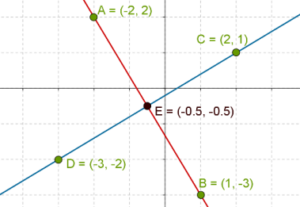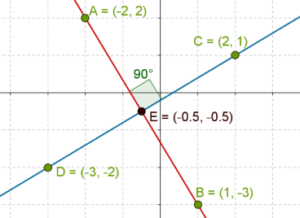# Relationships between Coefficients of Nature of Solutions

Go back to  'Pair of Linear Equations in Two Variables'

The question now is: given a pair of linear equations, can we figure out what kind of solution(s) it will have based on the coefficients. Let us state this problem more formally. Consider the following pair of linear equations:

$\begin{array}{l}{a_1}x + {b_1}y + {c_1} = 0\\{a_2}x + {b_2}y + {c_2} = 0\end{array}$

Using the coefficients in the two equations, can we determine whether this pair will have a unique solution, no solution or infinitely many solutions? Yes, we can. Let us understand how. For now, we will keep our discussion intuitive, but you will understand this with more rigor in a higher class.

Suppose that we rearrange and write the linear equations as follows:

\begin{align}&\left\{ \begin{array}{l}\frac{{{a_1}}}{{{b_1}}}x + y + \frac{{{c_1}}}{{{b_1}}} = 0\\\frac{{{a_2}}}{{{b_2}}}x + y + \frac{{{c_2}}}{{{b_2}}} = 0\end{array} \right.\\ &\Rightarrow \;\;\;\left\{ \begin{gathered}y = - \frac{{{a_1}}}{{{b_1}}}x - \frac{{{c_1}}}{{{b_1}}}\\y = - \frac{{{a_2}}}{{{b_2}}}x - \frac{{{c_2}}}{{{b_2}}}\end{gathered} \right.\end{align}

Compare these equations to the following linear equation:

$y = mx + b$

Recall that m is the slope of the line, that is, m determines the steepness of the line, while b is the y intercept and controls the vertical positioning of the line. Clearly, the slopes of the two lines above will be:

\begin{align}&{m_1} = - \frac{{{a_1}}}{{{b_1}}}\\&{m_2} = - \frac{{{a_2}}}{{{b_2}}}\end{align}

If we want the pair of linear equations to  have a unique solution, the slopes of the two lines should not be the same. This should be intuitively obvious. Thus, for a unique solution to exist, we must have:

\begin{align}&{m_1} \ne {m_2}\\&\Rightarrow \;\;\; - \frac{{{a_1}}}{{{b_1}}} \ne - \frac{{{a_2}}}{{{b_2}}}\\&\Rightarrow \;\;\;\frac{{{a_1}}}{{{b_1}}} \ne \frac{{{a_2}}}{{{b_2}}}\\&\Rightarrow \;\;\;\frac{{{a_1}}}{{{a_2}}} \ne \frac{{{b_1}}}{{{b_2}}}\end{align}

On the other hand, for no solution to exist, the lines must be parallel, which means that their slopes must be equal. Also, their y intercepts should not be the same; otherwise they will be identical lines. Thus, for no solution to exist, the condition on the coefficients will be:

\begin{align}&\left\{ \begin{array}{l}({\rm{i}})\;\;{m_1} = {m_2}\\ \Rightarrow \;\;\;\frac{{{a_1}}}{{{b_1}}} = \frac{{{a_2}}}{{{b_2}}}\;\; \Rightarrow \;\;\frac{{{a_1}}}{{{a_2}}} = \frac{{{b_1}}}{{{b_2}}}\{\rm{ii}})\;\;\; - \frac{{{c_1}}}{{{b_1}}} \ne - \frac{{{c_2}}}{{{b_2}}}\\ \Rightarrow \;\;\;\frac{{{b_1}}}{{{b_2}}} \ne \frac{{{c_1}}}{{{c_2}}}\end{array} \right.\\&\;\;\; \Rightarrow \;\;\;\frac{{{a_1}}}{{{a_2}}} = \frac{{{b_1}}}{{{b_2}}} \ne \frac{{{c_1}}}{{{c_2}}}\end{align} Finally, for the pair of linear equations to have infinitely many solutions, the two lines must be the same. This means that they should have the same slope, and their y intercepts should also be the same. Thus, the condition on the coefficients is: \begin{align}&\left\{ \begin{array}{l}({\rm{i}})\;\;\;{m_1} = {m_2}\\\;\;\; \Rightarrow \;\frac{{{a_1}}}{{{b_1}}} = \frac{{{a_2}}}{{{b_2}}} \Rightarrow \;\frac{{{a_1}}}{{{a_2}}} = \frac{{{b_1}}}{{{b_2}}}\\({\rm{ii}})\;\;\; - \frac{{{c_1}}}{{{b_1}}} = - \frac{{{c_2}}}{{{b_2}}}\\ \Rightarrow \;\;\;\frac{{{b_1}}}{{{b_2}}} = \frac{{{c_1}}}{{{c_2}}}\end{array} \right.\\&\;\; \Rightarrow \;\;\;\frac{{{a_1}}}{{{a_2}}} = \quad\frac{{{b_1}}}{{{b_2}}} = \frac{{{c_1}}}{{{c_2}}}\end{align} Note that this essentially means that one linear equation can be expressed as a multiple of the other. Only then can the constraint above be satisfied. When a linear pair of equations has one solution (intersecting lines) or infinitely many solutions (coincident lines), we say that it is a consistent pair. On the other hand, when a linear pair has no solution (parallel, non-coincident lines), we say that it is an inconsistent pair. Example 1: Consider the following pair of linear equations: $\begin{array}{l}5x + 3y + 4 = 0\\ - 3x + 5y + 1 = 0\end{array}$ Using the coefficients, check whether this pair is consistent. If it is, evaluate its solution graphically. Solution: We have: $\frac{5}{{ - 3}} \ne \frac{3}{5}$ This means that the given pair will have a unique solution, that is, it is a consistent pair. Now, we plot the lines corresponding to the two equations, and determine the point of intersection. In the following graph, we have plotted a pair of points for each line:Thus, the solution to this linear pair is $x = - \frac{1}{2},\;y = - \frac{1}{2}$ Example 2: Three linear equations are given below: \(\begin{array}{l}{\rm{I}}.\;\;\;\;2x - 3y + 1 = 0\\{\rm{II}}.\;\;\;7x + 4y - 3 = 0\\{\rm{III}}.\;\; - 4x + 6y + 5 = 0\end{array}

Identify the inconsistent pair(s) of equations.

Solution: I and II. We have:

$\frac{2}{7} \ne \frac{{ - 3}}{4}$

This pair is consistent and will have a unique solution.

II and III. We have:

$\frac{7}{{ - 4}} \ne \frac{4}{6}$

This pair is also consistent and will have a unique solution.

I and III. We have:

$\frac{2}{{ - 4}} = \frac{{ - 3}}{6} \ne \frac{1}{5}$

This pair is clearly inconsistent.

Example 3: Consider the following pair of linear equations:

$\begin{array}{l} 5x + 3y = - 4\\ - 3x + 5y = - 1 \end{array}$

a) Graphically determine the solution to this pair.

b) Using a protractor, measure the angle between the two lines.

c) Determine the product of the slopes of the two lines.

Solution:  We have already solved this pair a while back. The solution is:

$x = - \frac{1}{2},\;y = - \frac{1}{2}$

Below, we reproduce the graph once again, and using a protractor, we find that the two lines are perpendicular:Now, we calculate the slopes of the two lines by writing them as follows:

$\begin{array}{l}\left\{ \begin{array}{l}y = - \frac{5}{3}x - \frac{4}{3}\\y = \frac{3}{5}x - \frac{1}{5}\end{array} \right.\\ \Rightarrow \;\;\;{m_1} = - \frac{5}{3},\;{m_2} = \frac{3}{5}\end{array}$

The product of the two slopes is:

${m_1} \times {m_2} = - \frac{5}{3} \times \frac{3}{5} = 1$

This is an extremely important connection, which you will study in more detail later. When the product of the slopes of two lines corresponding to a pair of linear equations is $$- 1$$, the two lines will be perpendicular.

Pair of Linear Equations in 2 Variables
Pair of Linear Equations in 2 Variables
grade 10 | Questions Set 2
Pair of Linear Equations in 2 Variables
Pair of Linear Equations in 2 Variables
grade 10 | Questions Set 1